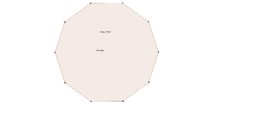•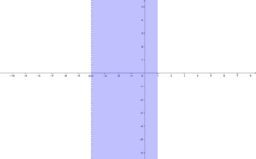### Inequality

Activity

•### Calculations

Activity

•### Calculations

Activity

•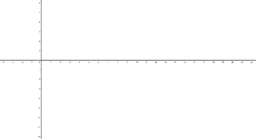### Calculations

Activity

•### Calculations

Activity

•### Calculations

Activity

•### Calculations

Activity

•### Calculations

Activity

•### Calculations

Activity

•### Calculations

Activity

•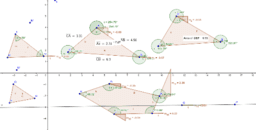### Angles

Activity

•### Effect of GeoGebra on Learning Outcomes of Maths Students

Book

•### Cumulative Frequency Curve

Activity

•### Sine & Cosine Graphs

Activity

•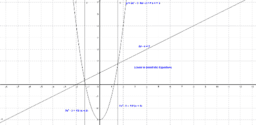Activity

•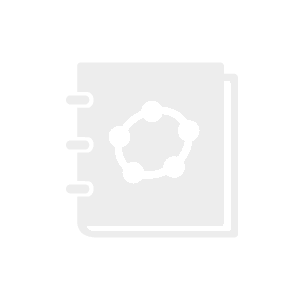### Application of GeoGebra into Teaching & Learning of Maths

Book

•### Cumulative Frequency Curve

Activity

••